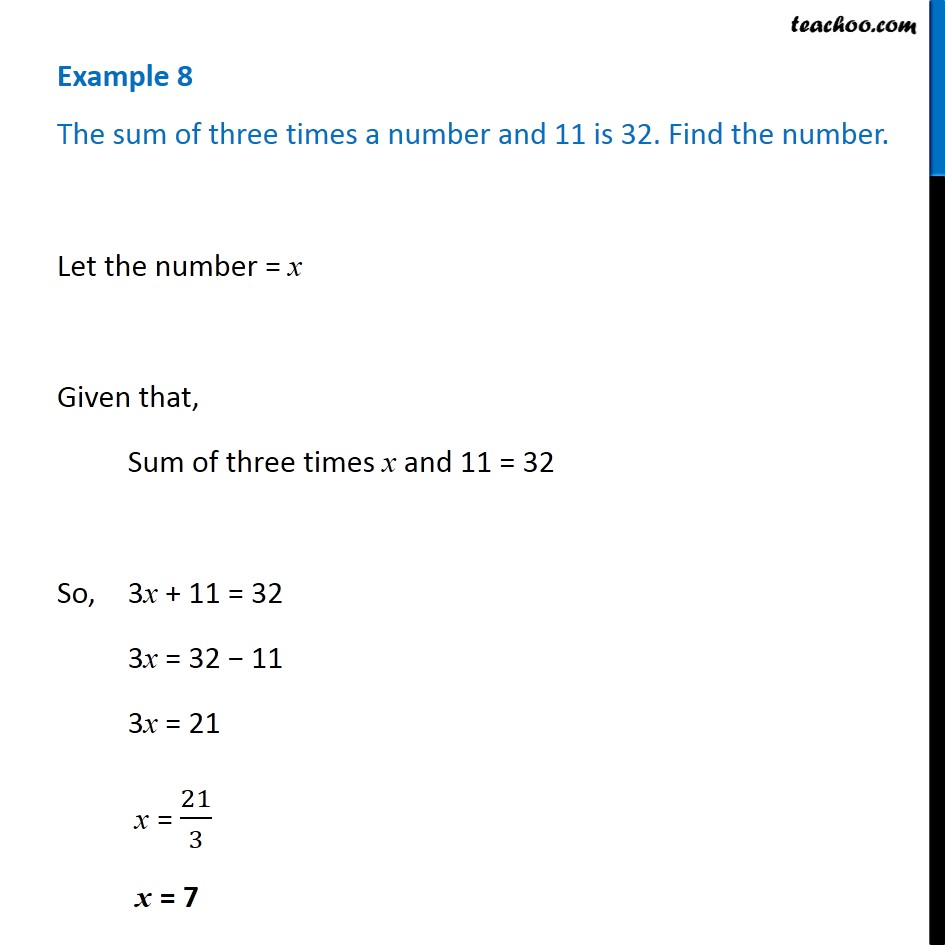Making equations from statements and solving

Chapter 4 Class 7 Simple Equations
Concept wiseIntroducing your new favourite teacher - Teachoo Black, at only ₹83 per month

### Transcript

Example 8 The sum of three times a number and 11 is 32. Find the number.Let the number = x Given that, Sum of three times x and 11 = 32 So, 3x + 11 = 32 3x = 32 − 11 3x = 21 x = 21/3 x = 7